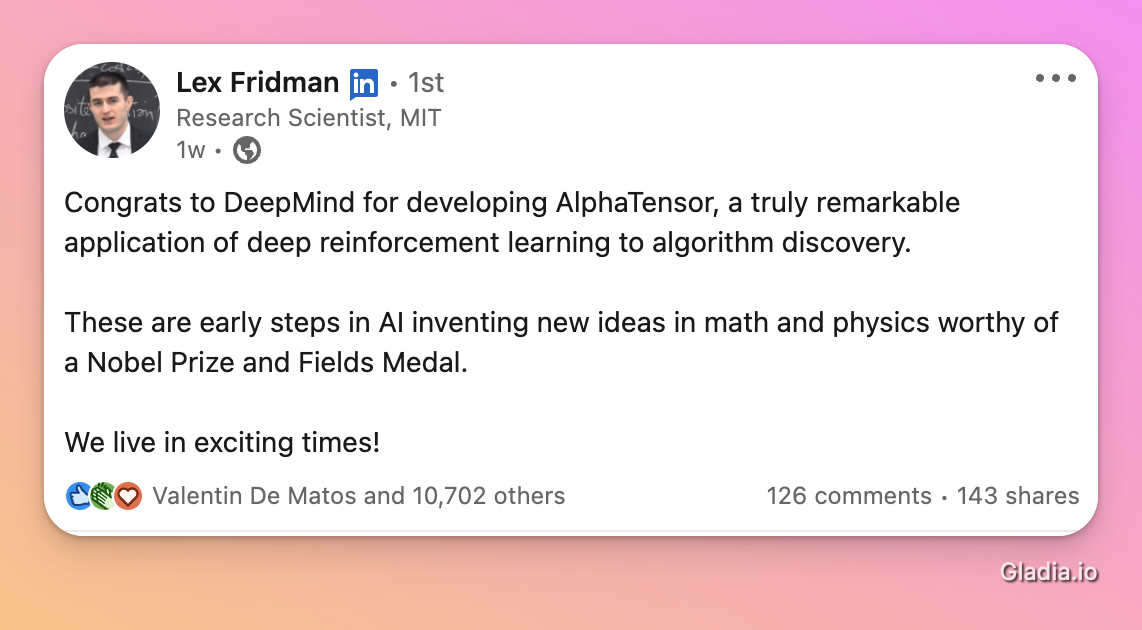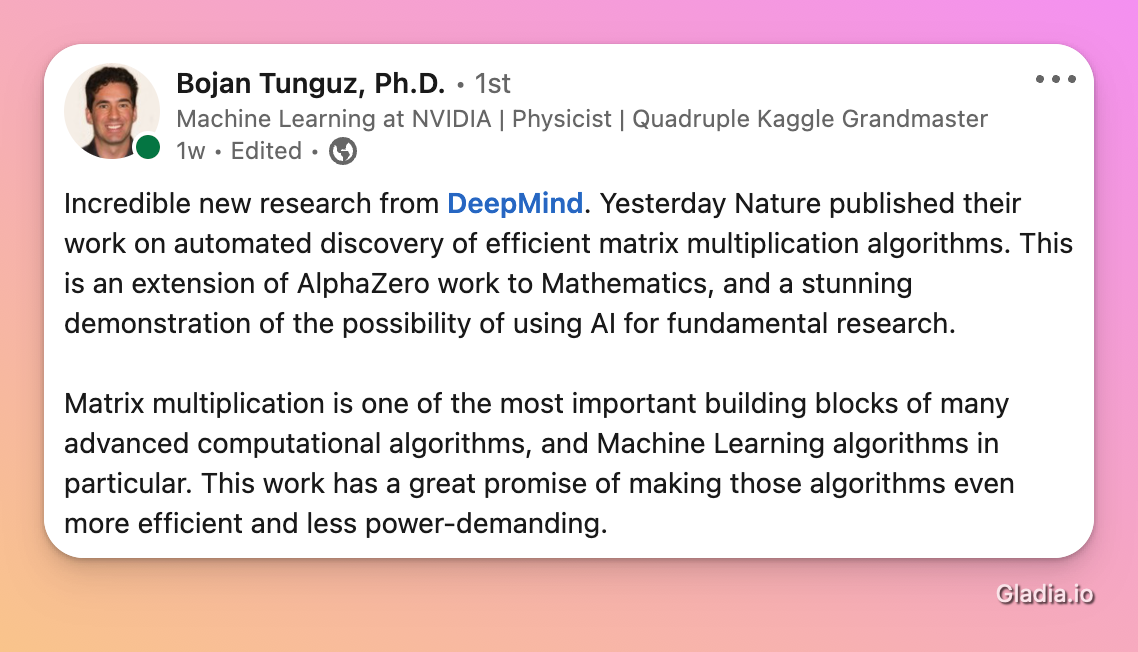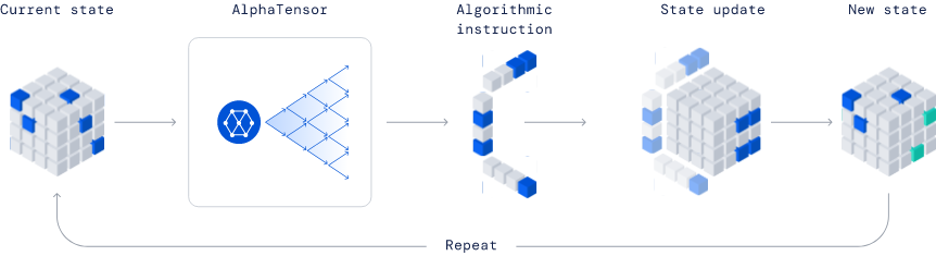Despite the prevalence of algorithms in today’s society, the process of discovering new ones is incredibly difficult. This is because humans are limited in what we can imagine and how to invent complex algorithms with our current scientific knowledge. DeepMind’s latest breakthrough, Alphatensor (published in Nature on October 5th) is groundbreaking because it circumvents these limitations.

# Introducing AlphaTensor

Although it is relatively easy to multiply two matrices, multiplying them quickly and correctly is not a trivial task. This is where matrix multiplication algorithms come in: they are sets of rules or equations that help computers perform matrix multiplication quickly.

There are many such algorithms, and some have been around for decades. However, researchers from DeepMind have recently shown that Artificial Intelligence (AI) techniques can discover more efficient methods for performing matrix multiplication than we might think of on our own.

# The Layman’s Curtain-Raiser to Matrix Multiplication and AlphaTensor

Mathematicians have long held the view that the conventional matrix multiplication algorithm is the most efficient, but German mathematician Volker Strassen startled the academic community in 1969 by demonstrating that there are actually better algorithms. His new method uses one less scalar multiplication when compared with the standard algorithm.

DeepMind has now leveraged AI to solve this classic problem by using cutting-edge techniques to find new algorithms, starting with matrix multiplication. AlphaTensor, a system that combines human intuition with deep learning models, uncovered new methods of multiplying matrices.

The significance of their discovery could feel “ordinary”, but what is unknown to many is that matrix multiplication is critical to the functioning of computers and other digital devices. This elementary math problem provides the foundation for everyday digital tasks like image processing, speech recognition, data compression, and many other functions.

The community got really excited about this announcement proving the AlphaTensor is a major milestone that has been acheived# And Now, The Technical Bit.

In 2015, Deepmind created a computer program that defeated a three-time world Go champion. The computer program used an AI technique refered to as reinforcement learning. The AI-enhanced software, AlphaGo, was even improved on in 2017 with AlphaZero.

The DeepMind researchers had to frame the matrix decomposition issue in a way that a model like AlphaZero could resolve. They found that by framing matrix decomposition as a single-player game (which they refer to as TensorGame), they could make it significantly related to the issues that AlphaZero had addressed.

The Deepmind team converted the problem of finding efficient algorithms for matrix multiplication into a single-player game. At each step of the game, the player selects how to combine different entries of the matrices to multiply. A score is assigned based on the number of selected operations required to reach the correct multiplication result.

Reinforcement learning techniques such as a reward system and penalties limit the number of moves the agent can make.

Like AlphaZero, AlphaTensor utilizes a deep neural network to direct a Monte Carlo tree search (MCTS) planning process. Taking a matrix as input, the network outputs a value and a policy that provides a distribution over potential actions. The value, however, furnishes an estimate of how the returns are distributed. In the concept of reinforcement learning, this return is the cumulative reward, starting from the current state.

In this game, a three-dimensional tensor (array of numbers) captures the distance between an incorrect algorithm and the correct one. The player can perform a set of allowed moves corresponding to algorithm instructions, with each move modifying the tensor to reduce its entities to zero. When the network succeeds at this task, it has proven that the algorithm is provably correct, and the number of steps it takes to zero out the tensor indicates how efficient that algorithm is.Alphatensor methodology. Image by DeepMind

AlphaTensor starts from the target tensor and uses the MCTS planner to choose the next action after each step. Finished games are used as feedback to improve the network parameters.

# The Bigger Picture: What Promise does AlphaTensor Hold?

Matrix multiplication algorithms are the bread and butter of the computing industry. In fact, many companies have invested huge amounts of resources into developing ways to optimize matrix multiplication. This is because any improvement to the efficiency of matrix multiplication is likely to have a widespread impact on the industry, potentially saving huge amounts of time and money for several companies.

Looking past matrix multiplication, the benefits for humanity is potentially endless. With such complex operations as the discovery of new algorithms made easy, there will be no limit to how much progress we can make in fields such as medicine, chemistry, and physics.

In addition to helping us solve problems faster or better than ever before, Alphatensor should also help guide further research in complexity theory, which aims to determine the fastest algorithms for solving computational problems.

# Takeaway

In most cases, designing an algorithm is a time-consuming and challenging process that requires a great deal of intuition and ingenuity. But with what can be referred to as the most notable breakthrough in algorithm discovery, the use of AI-designed algorithms to solve complex computational problems holds great promise.

Although it may seem counterintuitive, AI allows humans to design much better algorithms than before, as researchers from DeepMind showed. Their deep reinforcement learning-based matrix multiplication algorithm was more efficient than human-designed ones, which is a significant step forward in the field of algorithmic discovery.

# Going further

We recommend a few resources:

A very nice thread from Herum Shandilya